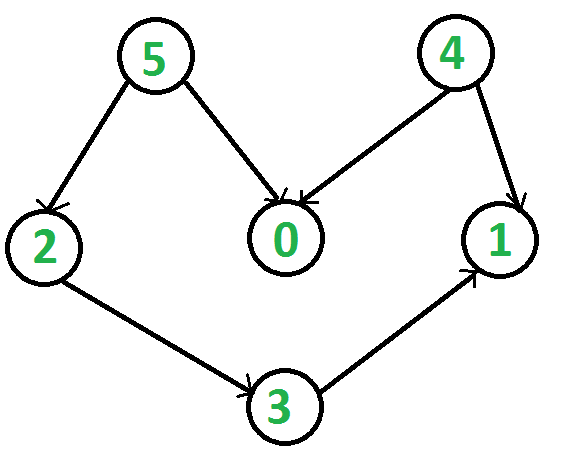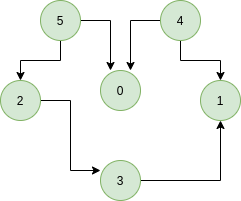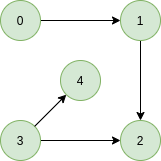# Kahn’s algorithm for Topological Sorting

Topological sorting for Directed Acyclic Graph (DAG) is a linear ordering of vertices such that for every directed edge uv, vertex u comes before v in the ordering. Topological Sorting for a graph is not possible if the graph is not a DAG.

For example, a topological sorting of the following graph is “5 4 2 3 1 0?. There can be more than one topological sorting for a graph. For example, another topological sorting of the following graph is “4 5 2 0 3 1″. The first vertex in topological sorting is always a vertex with in-degree as 0 (a vertex with no in-coming edges).Let’s look at few examples with proper explanation,
Example:

Input:Output: 5 4 2 3 1 0
Explanation: The topological sorting of a DAG is done in a order such that for every directed edge uv, vertex u comes before v in the ordering. 5 has no incoming edge. 4 has no incoming edge, 2 and 0 have incoming edge from 4 and 5 and 1 is placed at last.

Input:Output: 0 3 4 1 2
Explanation: 0 and 3 have no incoming edge, 4 and 1 has incoming edge from 0 and 3. 2 is placed at last.

## Recommended: Please solve it on “PRACTICE” first, before moving on to the solution.

A DFS based solution to find a topological sort has already been discussed.

Solution: In this article we will see another way to find the linear ordering of vertices in a directed acyclic graph (DAG). The approach is based on the below fact:

A DAG G has at least one vertex with in-degree 0 and one vertex with out-degree 0.
Proof: There’s a simple proof to the above fact is that a DAG does not contain a cycle which means that all paths will be of finite length. Now let S be the longest path from u(source) to v(destination). Since S is the longest path there can be no incoming edge to u and no outgoing edge from v, if this situation had occurred then S would not have been the longest path
=> indegree(u) = 0 and outdegree(v) = 0

Algorithm: Steps involved in finding the topological ordering of a DAG:
Step-1: Compute in-degree (number of incoming edges) for each of the vertex present in the DAG and initialize the count of visited nodes as 0.

Step-2: Pick all the vertices with in-degree as 0 and add them into a queue (Enqueue operation)

Step-3: Remove a vertex from the queue (Dequeue operation) and then.

1. Increment count of visited nodes by 1.
2. Decrease in-degree by 1 for all its neighboring nodes.
3. If in-degree of a neighboring nodes is reduced to zero, then add it to the queue.

Step 5: Repeat Step 3 until the queue is empty.

Step 5: If count of visited nodes is not equal to the number of nodes in the graph then the topological sort is not possible for the given graph.

How to find in-degree of each node?
There are 2 ways to calculate in-degree of every vertex:

1. Take an in-degree array which will keep track of
Traverse the array of edges and simply increase the counter of the destination node by 1.

```for each node in Nodes
indegree[node] = 0;
for each edge(src, dest) in Edges
indegree[dest]++```

Time Complexity: O(V+E)

2. Traverse the list for every node and then increment the in-degree of all the nodes connected to it by 1.
```    for each node in Nodes
If (list[node].size()!=0) then
for each dest in list
indegree[dest]++;```

Time Complexity: The outer for loop will be executed V number of times and the inner for loop will be executed E number of times, Thus overall time complexity is O(V+E).

The overall time complexity of the algorithm is O(V+E)

Below is C++ implementation of above algorithm. The implementation uses method 2 discussed above for finding indegrees.

## C++

 `// A C++ program to print topological ` `// sorting of a graph using indegrees. ` `#include ` `using` `namespace` `std; ` ` `  `// Class to represent a graph ` `class` `Graph { ` `    ``// No. of vertices' ` `    ``int` `V; ` ` `  `    ``// Pointer to an array containing ` `    ``// adjacency listsList ` `    ``list<``int``>* adj; ` ` `  `public``: ` `    ``// Constructor ` `    ``Graph(``int` `V); ` ` `  `    ``// Function to add an edge to graph ` `    ``void` `addEdge(``int` `u, ``int` `v); ` ` `  `    ``// prints a Topological Sort of ` `    ``// the complete graph ` `    ``void` `topologicalSort(); ` `}; ` ` `  `Graph::Graph(``int` `V) ` `{ ` `    ``this``->V = V; ` `    ``adj = ``new` `list<``int``>[V]; ` `} ` ` `  `void` `Graph::addEdge(``int` `u, ``int` `v) ` `{ ` `    ``adj[u].push_back(v); ` `} ` ` `  `// The function to do ` `// Topological Sort. ` `void` `Graph::topologicalSort() ` `{ ` `    ``// Create a vector to store ` `    ``// indegrees of all ` `    ``// vertices. Initialize all ` `    ``// indegrees as 0. ` `    ``vector<``int``> in_degree(V, 0); ` ` `  `    ``// Traverse adjacency lists ` `    ``// to fill indegrees of ` `    ``// vertices.  This step ` `    ``// takes O(V+E) time ` `    ``for` `(``int` `u = 0; u < V; u++) { ` `        ``list<``int``>::iterator itr; ` `        ``for` `(itr = adj[u].begin(); ` `             ``itr != adj[u].end(); itr++) ` `            ``in_degree[*itr]++; ` `    ``} ` ` `  `    ``// Create an queue and enqueue ` `    ``// all vertices with indegree 0 ` `    ``queue<``int``> q; ` `    ``for` `(``int` `i = 0; i < V; i++) ` `        ``if` `(in_degree[i] == 0) ` `            ``q.push(i); ` ` `  `    ``// Initialize count of visited vertices ` `    ``int` `cnt = 0; ` ` `  `    ``// Create a vector to store ` `    ``// result (A topological ` `    ``// ordering of the vertices) ` `    ``vector<``int``> top_order; ` ` `  `    ``// One by one dequeue vertices ` `    ``// from queue and enqueue ` `    ``// adjacents if indegree of ` `    ``// adjacent becomes 0 ` `    ``while` `(!q.empty()) { ` `        ``// Extract front of queue ` `        ``// (or perform dequeue) ` `        ``// and add it to topological order ` `        ``int` `u = q.front(); ` `        ``q.pop(); ` `        ``top_order.push_back(u); ` ` `  `        ``// Iterate through all its ` `        ``// neighbouring nodes ` `        ``// of dequeued node u and ` `        ``// decrease their in-degree ` `        ``// by 1 ` `        ``list<``int``>::iterator itr; ` `        ``for` `(itr = adj[u].begin(); ` `             ``itr != adj[u].end(); itr++) ` ` `  `            ``// If in-degree becomes zero, ` `            ``// add it to queue ` `            ``if` `(--in_degree[*itr] == 0) ` `                ``q.push(*itr); ` ` `  `        ``cnt++; ` `    ``} ` ` `  `    ``// Check if there was a cycle ` `    ``if` `(cnt != V) { ` `        ``cout << ``"There exists a cycle in the graph\n"``; ` `        ``return``; ` `    ``} ` ` `  `    ``// Print topological order ` `    ``for` `(``int` `i = 0; i < top_order.size(); i++) ` `        ``cout << top_order[i] << ``" "``; ` `    ``cout << endl; ` `} ` ` `  `// Driver program to test above functions ` `int` `main() ` `{ ` `    ``// Create a graph given in the ` `    ``// above diagram ` `    ``Graph g(6); ` `    ``g.addEdge(5, 2); ` `    ``g.addEdge(5, 0); ` `    ``g.addEdge(4, 0); ` `    ``g.addEdge(4, 1); ` `    ``g.addEdge(2, 3); ` `    ``g.addEdge(3, 1); ` ` `  `    ``cout << ``"Following is a Topological Sort of\n"``; ` `    ``g.topologicalSort(); ` ` `  `    ``return` `0; ` `} `

## Java

 `// A Java program to print topological ` `// sorting of a graph using indegrees ` `import` `java.util.*; ` ` `  `// Class to represent a graph ` `class` `Graph { ` `    ``// No. of vertices ` `    ``int` `V; ` ` `  `    ``// An Array of List which contains ` `    ``// references to the Adjacency List of ` `    ``// each vertex ` `    ``List adj[]; ` `    ``// Constructor ` `    ``public` `Graph(``int` `V) ` `    ``{ ` `        ``this``.V = V; ` `        ``adj = ``new` `ArrayList[V]; ` `        ``for` `(``int` `i = ``0``; i < V; i++) ` `            ``adj[i] = ``new` `ArrayList(); ` `    ``} ` ` `  `    ``// Function to add an edge to graph ` `    ``public` `void` `addEdge(``int` `u, ``int` `v) ` `    ``{ ` `        ``adj[u].add(v); ` `    ``} ` `    ``// prints a Topological Sort of the ` `    ``// complete graph ` `    ``public` `void` `topologicalSort() ` `    ``{ ` `        ``// Create a array to store ` `        ``// indegrees of all ` `        ``// vertices. Initialize all ` `        ``// indegrees as 0. ` `        ``int` `indegree[] = ``new` `int``[V]; ` ` `  `        ``// Traverse adjacency lists ` `        ``// to fill indegrees of ` `        ``// vertices. This step takes ` `        ``// O(V+E) time ` `        ``for` `(``int` `i = ``0``; i < V; i++) { ` `            ``ArrayList temp ` `                ``= (ArrayList)adj[i]; ` `            ``for` `(``int` `node : temp) { ` `                ``indegree[node]++; ` `            ``} ` `        ``} ` ` `  `        ``// Create a queue and enqueue ` `        ``// all vertices with indegree 0 ` `        ``Queue q ` `            ``= ``new` `LinkedList(); ` `        ``for` `(``int` `i = ``0``; i < V; i++) { ` `            ``if` `(indegree[i] == ``0``) ` `                ``q.add(i); ` `        ``} ` ` `  `        ``// Initialize count of visited vertices ` `        ``int` `cnt = ``0``; ` ` `  `        ``// Create a vector to store result ` `        ``// (A topological ordering of the vertices) ` `        ``Vector topOrder = ``new` `Vector(); ` `        ``while` `(!q.isEmpty()) { ` `            ``// Extract front of queue ` `            ``// (or perform dequeue) ` `            ``// and add it to topological order ` `            ``int` `u = q.poll(); ` `            ``topOrder.add(u); ` ` `  `            ``// Iterate through all its ` `            ``// neighbouring nodes ` `            ``// of dequeued node u and ` `            ``// decrease their in-degree ` `            ``// by 1 ` `            ``for` `(``int` `node : adj[u]) { ` `                ``// If in-degree becomes zero, ` `                ``// add it to queue ` `                ``if` `(--indegree[node] == ``0``) ` `                    ``q.add(node); ` `            ``} ` `            ``cnt++; ` `        ``} ` ` `  `        ``// Check if there was a cycle ` `        ``if` `(cnt != V) { ` `            ``System.out.println( ` `                ``"There exists a cycle in the graph"``); ` `            ``return``; ` `        ``} ` ` `  `        ``// Print topological order ` `        ``for` `(``int` `i : topOrder) { ` `            ``System.out.print(i + ``" "``); ` `        ``} ` `    ``} ` `} ` `// Driver program to test above functions ` `class` `Main { ` `    ``public` `static` `void` `main(String args[]) ` `    ``{ ` `        ``// Create a graph given in the above diagram ` `        ``Graph g = ``new` `Graph(``6``); ` `        ``g.addEdge(``5``, ``2``); ` `        ``g.addEdge(``5``, ``0``); ` `        ``g.addEdge(``4``, ``0``); ` `        ``g.addEdge(``4``, ``1``); ` `        ``g.addEdge(``2``, ``3``); ` `        ``g.addEdge(``3``, ``1``); ` `        ``System.out.println( ` `            ``"Following is a Topological Sort"``); ` `        ``g.topologicalSort(); ` `    ``} ` `} `

## Python

 `# A Python program to print topological sorting of a graph ` `# using indegrees ` `from` `collections ``import` `defaultdict ` ` `  `# Class to represent a graph ` `class` `Graph: ` `    ``def` `__init__(``self``, vertices): ` `        ``self``.graph ``=` `defaultdict(``list``) ``# dictionary containing adjacency List ` `        ``self``.V ``=` `vertices ``# No. of vertices ` ` `  `    ``# function to add an edge to graph ` `    ``def` `addEdge(``self``, u, v): ` `        ``self``.graph[u].append(v) ` ` `  ` `  `    ``# The function to do Topological Sort.  ` `    ``def` `topologicalSort(``self``): ` `         `  `        ``# Create a vector to store indegrees of all ` `        ``# vertices. Initialize all indegrees as 0. ` `        ``in_degree ``=` `[``0``]``*``(``self``.V) ` `         `  `        ``# Traverse adjacency lists to fill indegrees of ` `           ``# vertices.  This step takes O(V + E) time ` `        ``for` `i ``in` `self``.graph: ` `            ``for` `j ``in` `self``.graph[i]: ` `                ``in_degree[j] ``+``=` `1` ` `  `        ``# Create an queue and enqueue all vertices with ` `        ``# indegree 0 ` `        ``queue ``=` `[] ` `        ``for` `i ``in` `range``(``self``.V): ` `            ``if` `in_degree[i] ``=``=` `0``: ` `                ``queue.append(i) ` ` `  `        ``# Initialize count of visited vertices ` `        ``cnt ``=` `0` ` `  `        ``# Create a vector to store result (A topological ` `        ``# ordering of the vertices) ` `        ``top_order ``=` `[] ` ` `  `        ``# One by one dequeue vertices from queue and enqueue ` `        ``# adjacents if indegree of adjacent becomes 0 ` `        ``while` `queue: ` ` `  `            ``# Extract front of queue (or perform dequeue) ` `            ``# and add it to topological order ` `            ``u ``=` `queue.pop(``0``) ` `            ``top_order.append(u) ` ` `  `            ``# Iterate through all neighbouring nodes ` `            ``# of dequeued node u and decrease their in-degree ` `            ``# by 1 ` `            ``for` `i ``in` `self``.graph[u]: ` `                ``in_degree[i] ``-``=` `1` `                ``# If in-degree becomes zero, add it to queue ` `                ``if` `in_degree[i] ``=``=` `0``: ` `                    ``queue.append(i) ` ` `  `            ``cnt ``+``=` `1` ` `  `        ``# Check if there was a cycle ` `        ``if` `cnt !``=` `self``.V: ` `            ``print` `"There exists a cycle in the graph"` `        ``else` `: ` `            ``# Print topological order ` `            ``print` `top_order ` ` `  ` `  `g ``=` `Graph(``6``) ` `g.addEdge(``5``, ``2``); ` `g.addEdge(``5``, ``0``); ` `g.addEdge(``4``, ``0``); ` `g.addEdge(``4``, ``1``); ` `g.addEdge(``2``, ``3``); ` `g.addEdge(``3``, ``1``); ` ` `  `print` `"Following is a Topological Sort of the given graph"` `g.topologicalSort() ` ` `  `# This code is contributed by Neelam Yadav  `

Output:

```Following is a Topological Sort
4 5 2 0 3 1```

Complexity Analysis:

• Time Complexity: O(V+E).
The outer for loop will be executed V number of times and the inner for loop will be executed E number of times.
• Auxillary Space: O(V).
The queue needs to store all the vertices of the graph. So the space required is O(V)